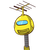# . In a class test containing 20 questions, every correct answer is awarded 5 marks, every incorrect answer is awarded

. In a class test containing 20 questions, every correct answer is awarded 5 marks, every incorrect answer is

awarded (-2) marks and 0 marks are awarded for every question not attempted. A student gets 6 questions

right and 4 questions wrong and leave 10 question.​

### 1 thought on “. In a class test containing 20 questions, every correct answer is awarded 5 marks, every incorrect answer is <br /><br />awarded”

1.= 5×6

= 30

=4×(-2)

= (-8)

Question not attempted = 10

= 10×0

= 0

Total Score = 30-8-0

= 22 marks